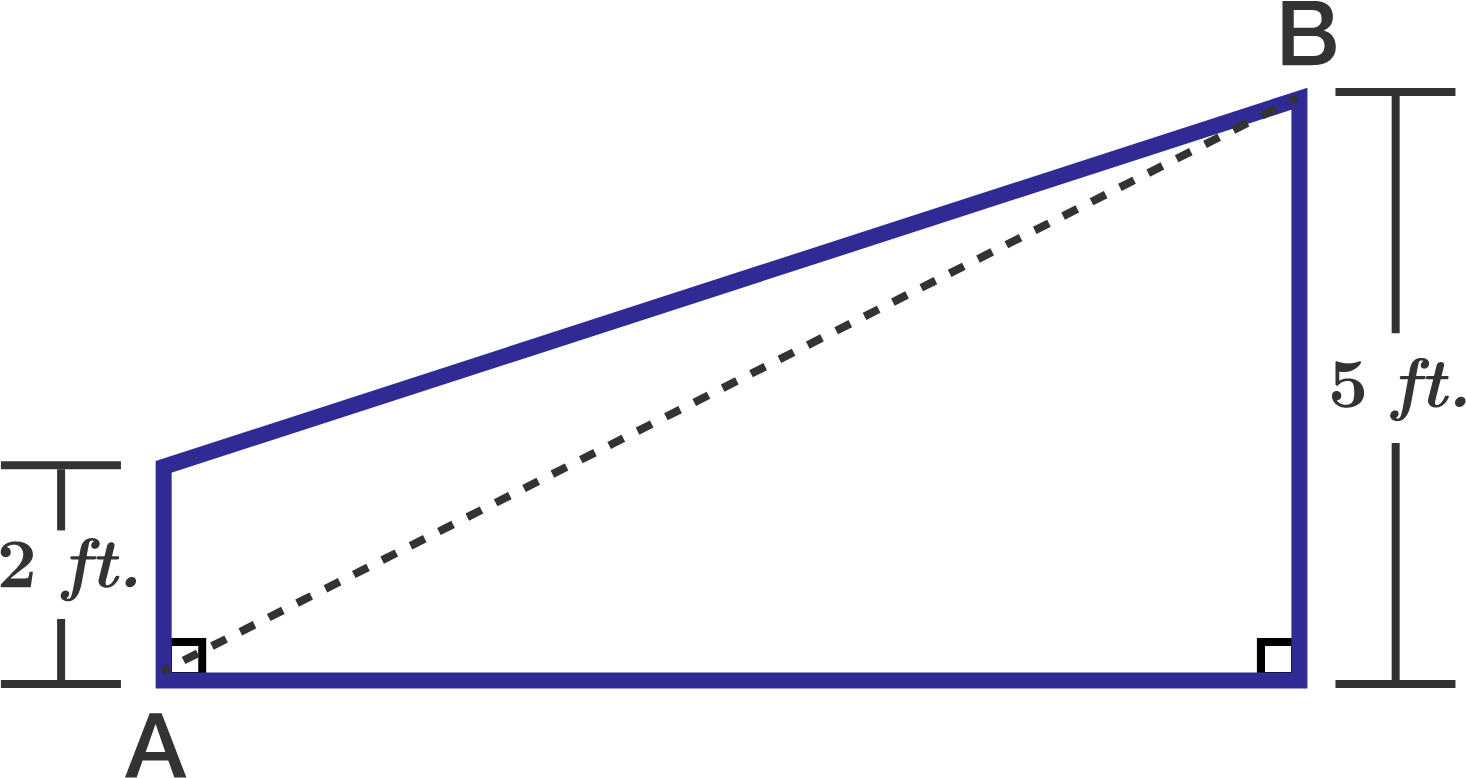# Cross Sectional Area

Geometry Level 1

The trapezoid shown in the given figure represents a cross section of the rudder of a ship.

If the distance from $A$ to $B$ is 13 feet, what is the area of the cross section of the rudder in square feet?×